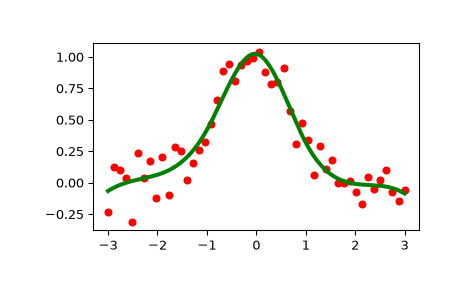# scipy.interpolate.LSQUnivariateSpline¶

class scipy.interpolate.LSQUnivariateSpline(x, y, t, w=None, bbox=[None, None], k=3, ext=0, check_finite=False)[source]

1-D spline with explicit internal knots.

Fits a spline y = spl(x) of degree k to the provided x, y data. t specifies the internal knots of the spline

Parameters
x(N,) array_like

Input dimension of data points – must be increasing

y(N,) array_like

Input dimension of data points

t(M,) array_like

interior knots of the spline. Must be in ascending order and:

```bbox < t < ... < t[-1] < bbox[-1]
```
w(N,) array_like, optional

weights for spline fitting. Must be positive. If None (default), weights are all equal.

bbox(2,) array_like, optional

2-sequence specifying the boundary of the approximation interval. If None (default), `bbox = [x, x[-1]]`.

kint, optional

Degree of the smoothing spline. Must be 1 <= k <= 5. Default is k = 3, a cubic spline.

extint or str, optional

Controls the extrapolation mode for elements not in the interval defined by the knot sequence.

• if ext=0 or ‘extrapolate’, return the extrapolated value.

• if ext=1 or ‘zeros’, return 0

• if ext=2 or ‘raise’, raise a ValueError

• if ext=3 of ‘const’, return the boundary value.

The default value is 0.

check_finitebool, optional

Whether to check that the input arrays contain only finite numbers. Disabling may give a performance gain, but may result in problems (crashes, non-termination or non-sensical results) if the inputs do contain infinities or NaNs. Default is False.

Raises
ValueError

If the interior knots do not satisfy the Schoenberg-Whitney conditions

`UnivariateSpline`

a smooth univariate spline to fit a given set of data points.

`InterpolatedUnivariateSpline`

a interpolating univariate spline for a given set of data points.

`splrep`

a function to find the B-spline representation of a 1-D curve

`splev`

a function to evaluate a B-spline or its derivatives

`sproot`

a function to find the roots of a cubic B-spline

`splint`

a function to evaluate the definite integral of a B-spline between two given points

`spalde`

a function to evaluate all derivatives of a B-spline

Notes

The number of data points must be larger than the spline degree k.

Knots t must satisfy the Schoenberg-Whitney conditions, i.e., there must be a subset of data points `x[j]` such that `t[j] < x[j] < t[j+k+1]`, for `j=0, 1,...,n-k-2`.

Examples

```>>> from scipy.interpolate import LSQUnivariateSpline, UnivariateSpline
>>> import matplotlib.pyplot as plt
>>> rng = np.random.default_rng()
>>> x = np.linspace(-3, 3, 50)
>>> y = np.exp(-x**2) + 0.1 * rng.standard_normal(50)
```

Fit a smoothing spline with a pre-defined internal knots:

```>>> t = [-1, 0, 1]
>>> spl = LSQUnivariateSpline(x, y, t)
```
```>>> xs = np.linspace(-3, 3, 1000)
>>> plt.plot(x, y, 'ro', ms=5)
>>> plt.plot(xs, spl(xs), 'g-', lw=3)
>>> plt.show()
```Check the knot vector:

```>>> spl.get_knots()
array([-3., -1., 0., 1., 3.])
```

Constructing lsq spline using the knots from another spline:

```>>> x = np.arange(10)
>>> s = UnivariateSpline(x, x, s=0)
>>> s.get_knots()
array([ 0.,  2.,  3.,  4.,  5.,  6.,  7.,  9.])
>>> knt = s.get_knots()
>>> s1 = LSQUnivariateSpline(x, x, knt[1:-1])    # Chop 1st and last knot
>>> s1.get_knots()
array([ 0.,  2.,  3.,  4.,  5.,  6.,  7.,  9.])
```

Methods

 `__call__`(x[, nu, ext]) Evaluate spline (or its nu-th derivative) at positions x. Construct a new spline representing the antiderivative of this spline. `derivative`([n]) Construct a new spline representing the derivative of this spline. Return all derivatives of the spline at the point x. Return spline coefficients. Return positions of interior knots of the spline. Return weighted sum of squared residuals of the spline approximation. `integral`(a, b) Return definite integral of the spline between two given points. Return the zeros of the spline. Continue spline computation with the given smoothing factor s and with the knots found at the last call.
 validate_input